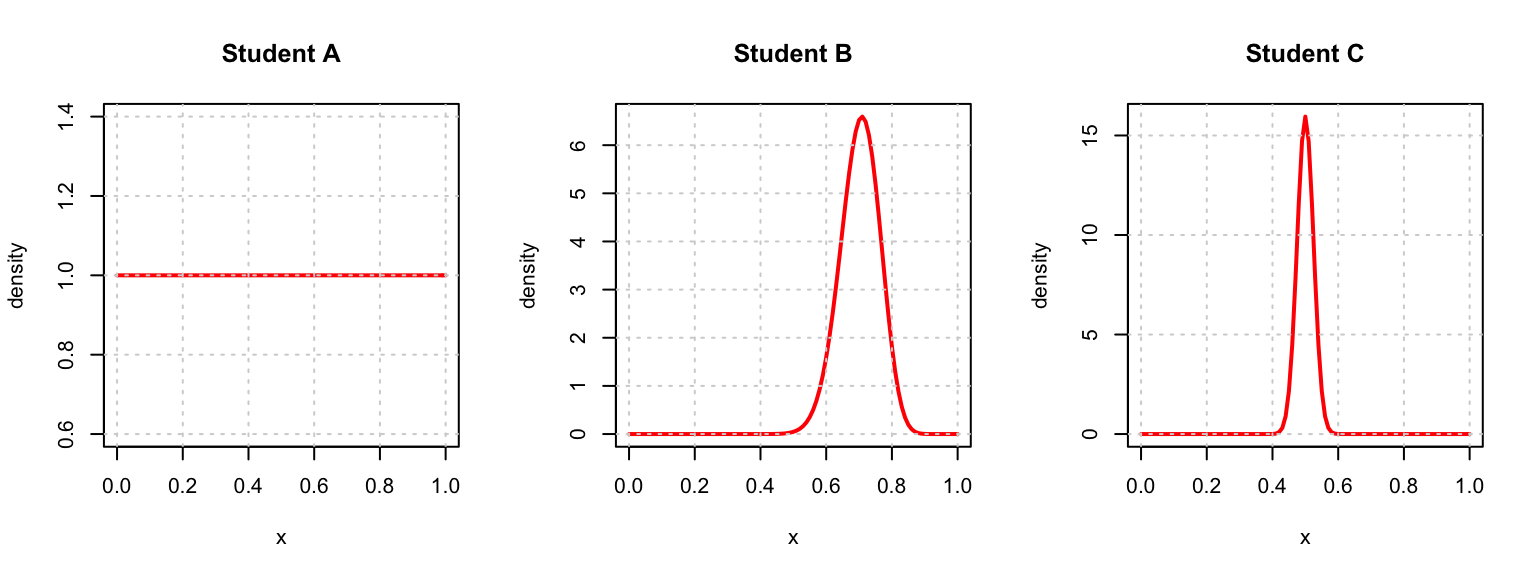For this assignment we will be interested in $$\theta$$, the pass completion percentage of Dwayne Haskins, the starting quarterback of The Ohio State Buckeyes.

At the beginning of the 2018 season three college students state their prior belief about $$\theta$$.

• Student A, a student from UIUC, knows literally nothing about football and uses a very uninformative prior, a Beta distribution with $$\alpha = 1$$ and $$\beta = 1$$.
• Student B is a clever OSU fan and decides to use Haskins’ 2017 statistics to inform their prior, thus they use a Beta distribution with $$\alpha = 40$$ and $$\beta = 17$$.
• Student C is a Xichigan fan, and has a strong belief that Haskins has a completion percentage of 50%, thus uses a Beta distribution with $$\alpha = 200$$ and $$\beta = 200$$.As of the writing of this assignment (before Saturday’s game, last semester), Haskins has completed 294 of 424 passes during the 2018 season. Use this as the observed data. All three students will use a Bernoulli likelihood for this data when updating their beliefs.

# Exercise 1

Use the above information to calculate an estimate of $$\theta$$ for each student. Call the estimates $$\hat{\theta}_A$$, $$\hat{\theta}_B$$, and $$\hat{\theta}_C$$. (Use the mean of the posterior distribution for each student as their estimator.) Hint: Recall the mean of a beta distribution.

# Exercise 2

Calculate a 90% credible interval for $$\theta$$ from student A. Hint: qbeta() will be helpful. If you use R please supply the line(s) of code used.

# Exercise 3

Calculate a 95% credible interval for $$\theta$$ from student B. Hint: qbeta() will be helpful. If you use R please supply the line(s) of code used.

# Exercise 4

Calculate a 99% credible interval for $$\theta$$ from student C. Hint: qbeta() will be helpful. If you use R please supply the line(s) of code used.

# Exercise 5

Assume you are Student B. Use your prior, and the observed data, to test $$H_0: \theta > 0.73$$ vs $$H_1: \theta \leq 0.73$$. Hint: pbeta() will be helpful. If you use R please supply the line(s) of code used.